Maths Brain Teasers 100 :: Can You - Complete The Puzzle?Hey All; @StemGeeks Mathematician;

Without further, ado, let's get to our puzzle for today:: Maths Brain Teasers 100 :: Can You - Complete The Puzzle?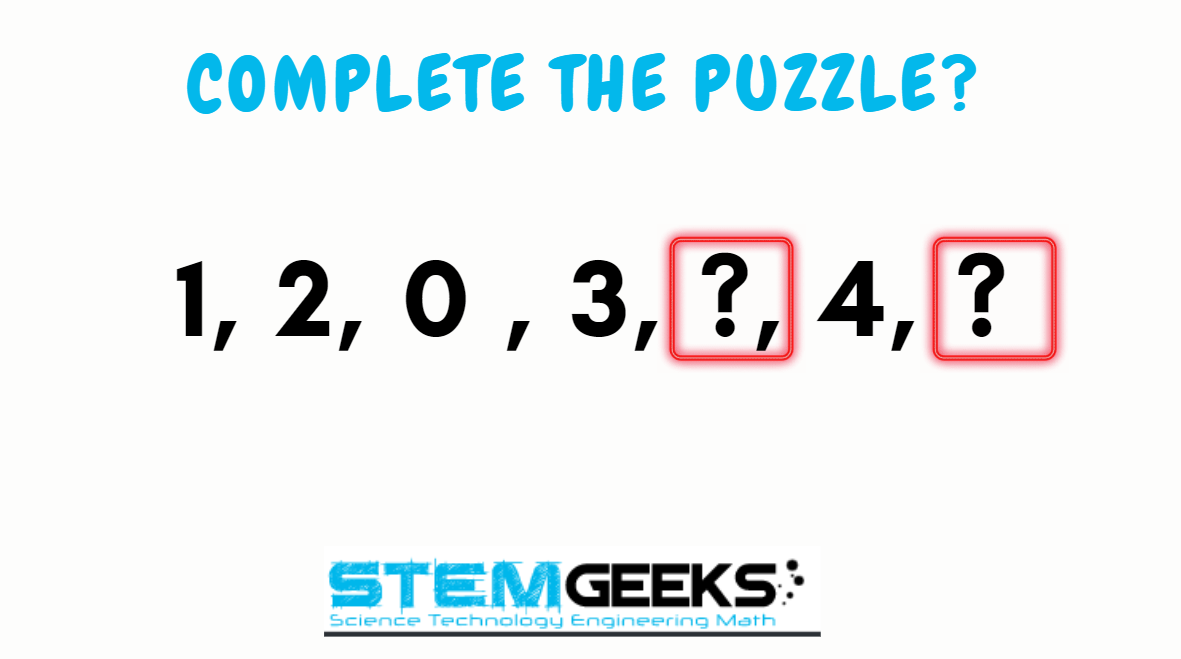Have a close look at the image given and see if you can decode the logic given in the image to complete the puzzle. Try finding out the right NUMBER that Simplifies or Decodes the logic to the given puzzle. Replace the question marks with the right number.

There is logic to resolve the puzzle; try finding out the logic and it should be resolved in seconds.. Guesswork isn't going to help...

With that, I'll leave you all with the Maths Brain Teasers 100 :: Can You - Complete The Puzzle? Good luck solving the puzzle...

Maths Brain Teasers 99 :: Can You - Complete The Sequence? - Solved with Explanation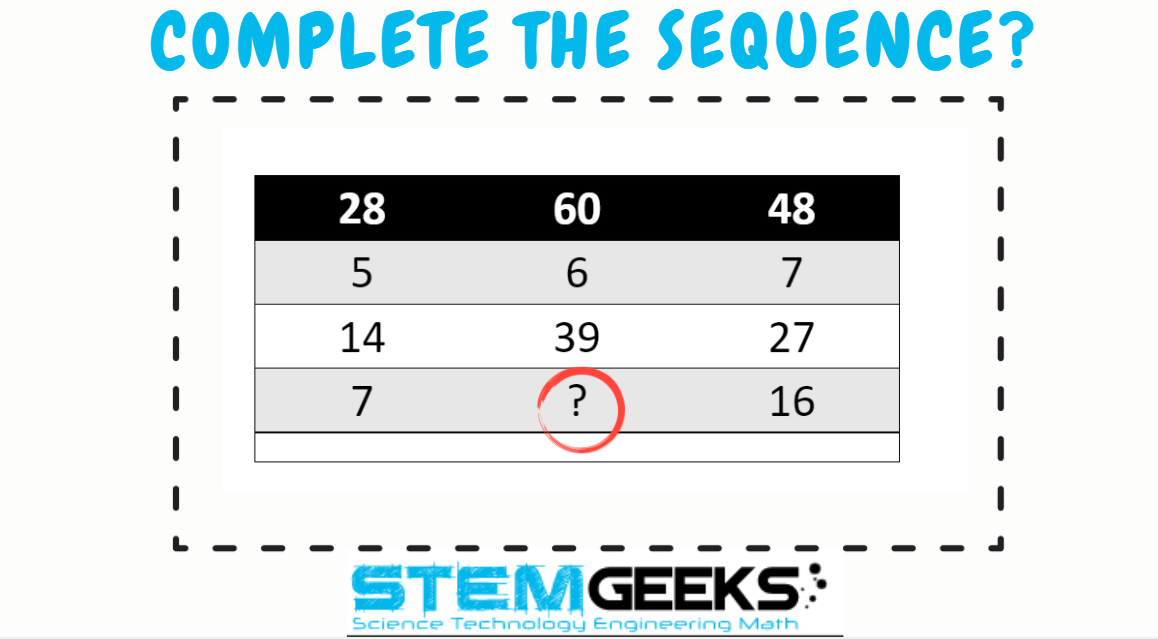Let's have a look at the Step by Step instructions to Solve the Maths Brain Teasers 99 :: Can You - Complete The Sequence?

If you carefully look at the Image in question; You would have noticed the following pattern is being followed::

The logic was::

The number in the second column is THREE times the difference between the numbers in the third and first columns

Let's take the example 1::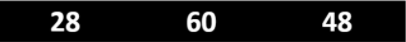X = 3 * 20 = 60

Now, let's take ROW in question::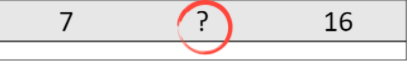X = 3 * 9 = 27

Hence the answer to the Puzzle is Number:: 27

STEM token GiveAway

I'll be again doing a giveaway of STEM tokens to the lucky random winner with the correct answer. For the last contest, which was Maths Brain Teasers 99 :: Can You - Complete The Sequence?.

We had only 1 entry and I am happy to see the engagement. At the same time, it's great to see the detailed answers provided by everyone as to how they arrived at the solution of the puzzle.. Well done guys.. Way to GO... Now the results part; @codingdefined provided the right answer and hence he is the clear WINNER of this puzzle No:: 99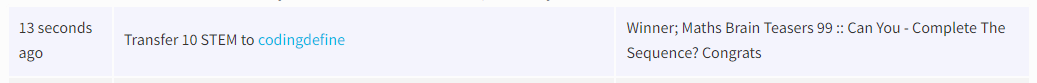Congratulations @codingdefined - You WIN yourself 10 STEM tokens. You should be having the rewards in your STEM Wallet Soon.

Math Quote for the Day::

Here is the motivation to solve the Maths Equation Puzzle?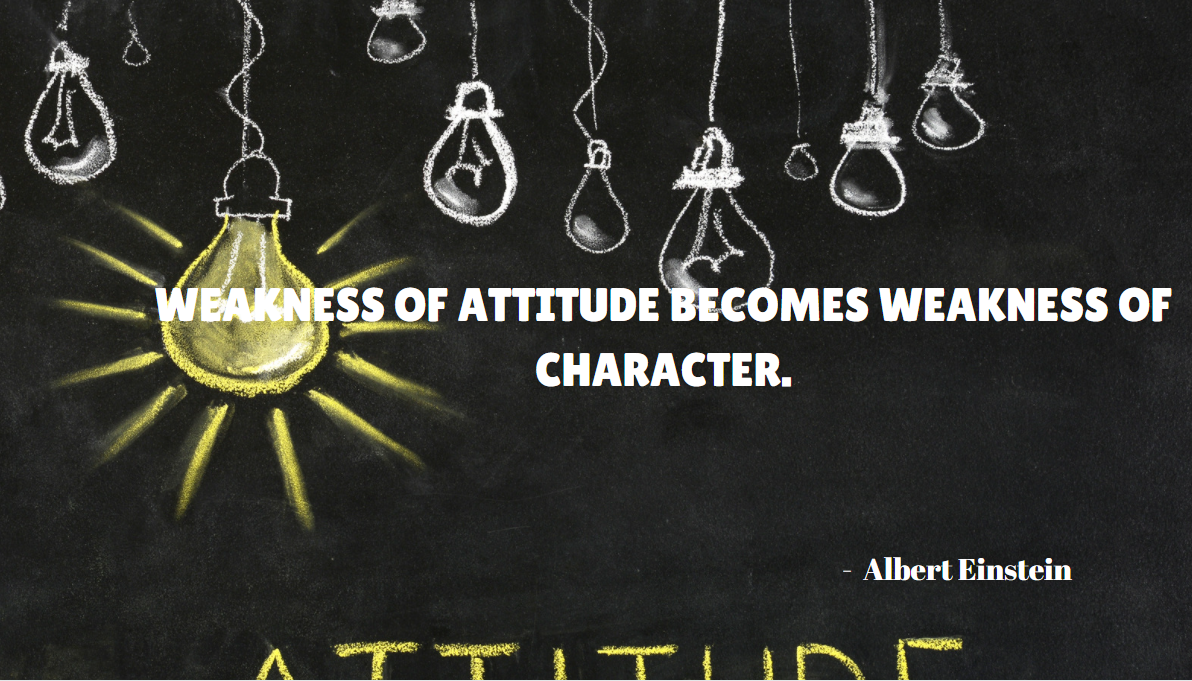If you like my work, then please spread the Word.. that we do have the Math Brain Teasers competition here @StemGeeks platform. Reblog is much appreciated.

Best RegardsFind Me on the Other Social Media Platforms::PS:: All the Maths Brain Teasers; are made by me using the Pro Canva License Version

0
0
0.000I think -1 and -2. Because the 2 3 4 are increasing by 1 and 1 0 -1 -2 will be decreasing by 1

0
0
0.0002 alternative series are here:
1,0,-1,-2
2,3,4

0
0
0.000Hi, i think th esequence is:
1,2,0,3,-1,4,-2
The calculation formula being to take the 3 previous numbers, add the first two and subtract the third.

0
0
0.000